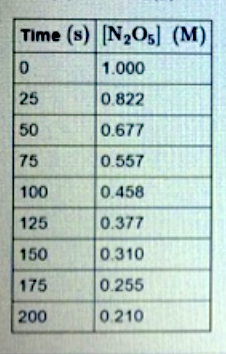# Problem: The data below show the concentration of N 2O5 versus time for the following reaction: N2O5 (g) → NO3 (g) + NO2 (g) Assuming the reaction is first order, determine the value of the rate constant. Predict the concentration of N2O5 at 300 s.

###### FREE Expert Solution###### Problem Details

The data below show the concentration of N 2O5 versus time for the following reaction:

N2O5 (g) → NO3 (g) + NO2 (g)

Assuming the reaction is first order, determine the value of the rate constant.Predict the concentration of N2O5 at 300 s.

What scientific concept do you need to know in order to solve this problem?

Our tutors have indicated that to solve this problem you will need to apply the Integrated Rate Law concept. You can view video lessons to learn Integrated Rate Law. Or if you need more Integrated Rate Law practice, you can also practice Integrated Rate Law practice problems.

What is the difficulty of this problem?

Our tutors rated the difficulty ofThe data below show the concentration of N 2O5 versus time f...as high difficulty.

How long does this problem take to solve?

Our expert Chemistry tutor, Dasha took 8 minutes and 29 seconds to solve this problem. You can follow their steps in the video explanation above.

What professor is this problem relevant for?

Based on our data, we think this problem is relevant for Professor Timkovich's class at UA.